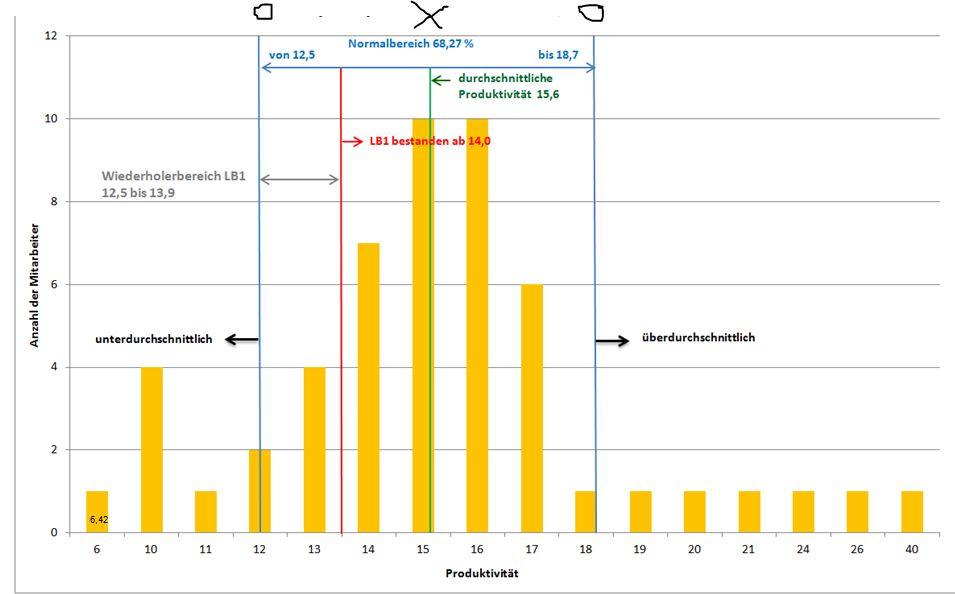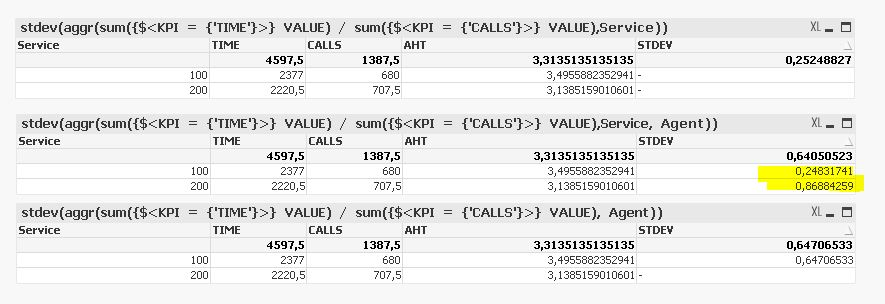# QlikView App Development

Discussion Board for collaboration related to QlikView App Development.

HighlightedNot applicable

## STDEV() and Set Analysis

Hello Community,

i have a Table that containes values for ACD-Data like this:

SERVICE   AGENT   KPI         VALUE

100               1          calls          20

100               2          calls          15

100               3          calls          30

200               1          time          60

200               2          time          42

200               3          time          81

Then there is a formula with set analysis, that creates the average time per call

sum({\$<KPI = {'time'}>} VALUE) / sum({\$<KPI = {'calls'}>} VALUE)

Now i need to build the STDEV for the average time per call. Obviusly simply putting the above formula into the stdev as an argument doens't do the trick. Any Ideas how to solve this without having to remodel the tabel or precalculate the KPI?

Thanks for suggestions and hints if not solutionsBest,

Hannes

Tags (2)
1 Solution

Accepted SolutionsEmployee

## Re: STDEV() and Set Analysis

Let's see if I get this straight....

One service has many agents; one agent makes many calls.

In your chart, you have Anzahl der Mitarbeiter as Y-axis. That means that you have calculated the average call time per person, per agent. Right? One value per agent and service. (Many calls are collapsed into one value.) This is calculated through

=Sum({\$<KPI = {'time'}>} VALUE) / Sum({\$<KPI = {'calls'}>} VALUE)

And then you want to calculate the Stdev to find the Normalbereich, by looking how the above value varies within a service. Then you want to loop over the previous calculation and calculate one value per service. (Many agents are collapsed into one value.) Right? Then you should use

=Stdev(Aggr(Sum({\$<KPI = {'time'}>} VALUE) / Sum({\$<KPI = {'calls'}>} VALUE),SERVICE,AGENT))

The Aggr() will loop over all combinations of SERVICE and AGENT. Then you should display this in a chart with SERVICE as dimension. The Stdev() function will then collaps several AGENTS into one value, which is what you need.

HIC

See Average – Which average? about internal grouping entities.

15 Replies
Valued Contributor

## Re: STDEV() and Set Analysis

Hi!

You can use aggr.

stdev(aggr(sum({\$<KPI = {'time'}>} VALUE) / sum({\$<KPI = {'calls'}>} VALUE),Agent))Employee

## Re: STDEV() and Set Analysis

It depends...

You say you want to calculate the "STDEV for the average time per call" - i.e. you want to see how the call time varies within the same service and agent. My answer is that this is not possible, given the data. You don't have that information. You need a table with the times for the individual calls to calculate this.

However, if you want to see how the average time varies between services and agents, then you can use Aggr(). But you need both service and agent as grouping symbols in the Aggr():

Stdev(Aggr(Sum({\$<KPI = {'time'}>} VALUE) / Sum({\$<KPI = {'calls'}>} VALUE),SERVICE,AGENT))

HICNot applicable

## Re: STDEV() and Set Analysis

Hi Sergey,

thanks very much for the formula. That's the way to go.

HIC is right about the dimensons. Agent alone isn't sufficent. But i wasn't very precise with describing my goal, so sorry for that.

Best

HannesNot applicable

## Re: STDEV() and Set Analysis

Hi Henric,

thanks a lot. You are right, i didn't make myself clear here.

And i made a mistake in the table aswell. All required data to calculate the average time per call grouped by service, be it 100 or 200, is available. So i can generate the average time per call (average handling time AHT) via Sum({\$<KPI = {'time'}>} VALUE) / Sum({\$<KPI = {'calls'}>} VALUE) for each individual service as a dimension in say a bar chart or pivot table.

I need the stdev for all calls within a service, i dont need the information for a single agent

The goal is to determine the range of average handling times for each service which represent 68% of the agents, more specific the bottom value of average handling time. So what i want do do with the value returned by the stdev() formula is to add it to and substract it from the avergage, thus getting the range of times that is representing 68% of the agents.I dont want to build this chart, it's just to illustrate where i am aiming at. I just need the numbers. The green line (marked with x on top) is calculated by time / calls. The blue line should be calculated by (time / calls) - stdev(time/calls). The chart data is from one service, and the kpi here is calls / time - the mechanism should be the same.

That seems to be the second part of your answer, if i am getting you correctly.

The formula works and is given me data. I am just a little bit confused how i need to group correctly, to geht the data that i want.

Best HannesEmployee

## Re: STDEV() and Set Analysis

Let's see if I get this straight....

One service has many agents; one agent makes many calls.

In your chart, you have Anzahl der Mitarbeiter as Y-axis. That means that you have calculated the average call time per person, per agent. Right? One value per agent and service. (Many calls are collapsed into one value.) This is calculated through

=Sum({\$<KPI = {'time'}>} VALUE) / Sum({\$<KPI = {'calls'}>} VALUE)

And then you want to calculate the Stdev to find the Normalbereich, by looking how the above value varies within a service. Then you want to loop over the previous calculation and calculate one value per service. (Many agents are collapsed into one value.) Right? Then you should use

=Stdev(Aggr(Sum({\$<KPI = {'time'}>} VALUE) / Sum({\$<KPI = {'calls'}>} VALUE),SERVICE,AGENT))

The Aggr() will loop over all combinations of SERVICE and AGENT. Then you should display this in a chart with SERVICE as dimension. The Stdev() function will then collaps several AGENTS into one value, which is what you need.

HIC

See Average – Which average? about internal grouping entities.Not applicable

## Re: STDEV() and Set Analysis

Hi Henric,

an absolutly perfect description of what i want to do.

The last bit i don't understand is the following:

When i use a pivot chart and have Service as the only dimension i get theese results for the formula, depending on the way i group (grouping displayed in chart title - the result in the middle is what i am aiming at)

To get a value for each service i need to group over service and agentHaving read your article (thx a lot - great piece of work) i thought that grouping by service is what i need to do -  as you suggested.

But this isn't giving me one value for each service and a different value as well.

Having grouped by agent in the third object displays, that the aggr won't consider the chart dimensions.

But still, why do i need to aggregate by agent and service, though agent isn't a dimension at all.

I can't comprehend why...  Maybe you allow me once more to tap into your vast scope of qlikview know how ...

Best Regards

HannesMVP

## Re: STDEV() and Set Analysis

Since you show count of 'Mitarbeiter' (Agents?) in above sample graph, I would assume that you want a value of productivity (calls / time)  per 'Mitarbeiter'. Not sure how service comes into play here.

You seem to have service as an independent variable, so maybe just use it as a filter? I don't see 'service' appearing somewhere in your sample chart.

Basically, I think you need to step back and consider to what finest granularity you need to aggregate your productivity (i.e. aggregate calls / time values) to get the basic KPI, the average and standard deviation.

What I mean, create a table with a column that shows the single productivity values. If you got the table, finding the average and standard deviation  is simple.Employee

## Re: STDEV() and Set Analysis

You are of course right. My answer above contained an error (which I now have corrected). The Aggr() should use both SERVICE and AGENT as dimensions, and your char should just have SERVICE as dimension. So your middle chart is indeed the correct one.

HICNot applicable

## Re: STDEV() and Set Analysis

Hi swuehl,

thx for your suggestion. I don't want to create neither the productivity nor the average handling time within the script. It would lead us a bit far trying to explain why in detail, but this would simply deviate from the way the data is structured throughout the application.

Hence the formula above is the path laid out before me.

The chart i posted shows what happens within a service. A service is one of many phonenumbers we handle in our ACD. So yes, Agent is the right term. Anyway, I need to display the average handling time and/or productivity for each service.

See the charts in my former reply to Henrics post. That is what i want to achieve.

Best

Hannes